# Geometrical Meaning of Differentiation Smallness

It is useful to consider what geometrical meaning can be given to the differential coefficient.

In the first place, any function of $x$, such, for example, as $x^2$, or $\sqrt{x}$, or $ax+b$, can be plotted as a curve; and nowadays every schoolboy is familiar with the process of curve-plotting.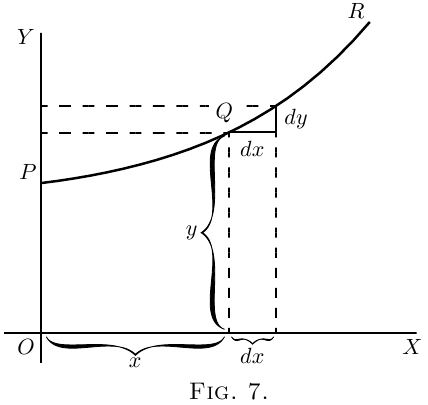Let $PQR$, in Figure 7, be a portion of a curve plotted with respect to the axes of coordinates $OX$ and $OY$. Consider any point $Q$ on this curve, where the abscissa of the point is $x$ and its ordinate is $y$. Now observe how $y$ changes when $x$ is varied. If $x$ is made to increase by a small increment $dx$, to the right, it will be observed that $y$ also (in this particular curve) increases by a small increment $dy$ (because this particular curve happens to be an ascending curve). Then the ratio of $dy$ to $dx$ is a measure of the degree to which the curve is sloping up between the two points $Q$ and $T$. As a matter of fact, it can be seen on the figure that the curve between $Q$ and $T$ has many different slopes, so that we cannot very well speak of the slope of the curve between $Q$ and $T$. If, however, $Q$ and $T$ are so near each other that the small portion $QT$ of the curve is practically straight, then it is true to say that the ratio $\dfrac{dy}{dx}$ is the slope of the curve along $QT$. The straight line $QT$ produced on either side touches the curve along the portion $QT$ only, and if this portion is indefinitely small, the straight line will touch the curve at practically one point only, and be therefore a tangent to the curve.

This tangent to the curve has evidently the same slope as $QT$, so that $\dfrac{dy}{dx}$ is the slope of the tangent to the curve at the point $Q$ for which the value of $\dfrac{dy}{dx}$ is found.

We have seen that the short expression “the slope of a curve” has no precise meaning, because a curve has so many slopes–in fact, every small portion of a curve has a different slope. “The slope of a curve at a point” is, however, a perfectly defined thing; it is the slope of a very small portion of the curve situated just at that point; and we have seen that this is the same as “the slope of the tangent to the curve at that point.”

Observe that $dx$ is a short step to the right, and $dy$ the corresponding short step upwards. These steps must be considered as short as possible–in fact indefinitely short,–though in diagrams we have to represent them by bits that are not infinitesimally small, otherwise they could not be seen.

We shall hereafter make considerable use of this circumstance that $\dfrac{dy}{dx}$ represents the slope of the curve at any point.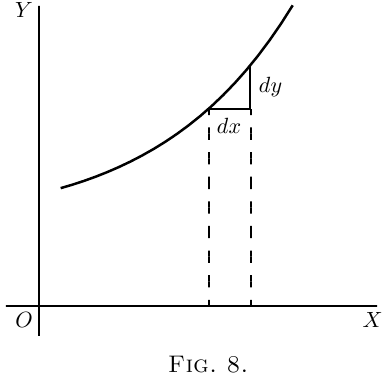If a curve is sloping up at $45°$ at a particular point, as in Figure 8, $dy$ and $dx$ will be equal, and the value of $\dfrac{dy}{dx} = 1$.

If the curve slopes up steeper than $45°$ (Figure 9), $\dfrac{dy}{dx}$ will be greater than $1$.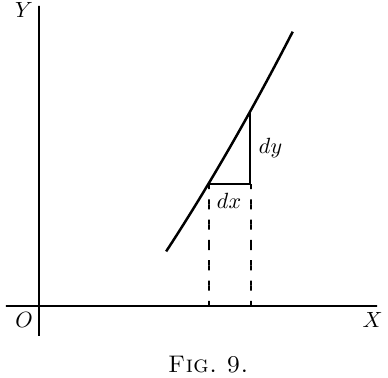If the curve slopes up very gently, as in Figure 10, $\dfrac{dy}{dx}$ will be a fraction smaller than $1$.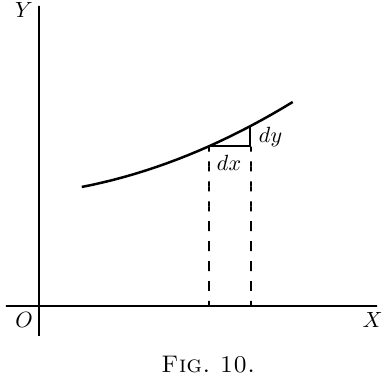If a curve slopes downward, as in Figure 11, $dy$ will be a step down, and must therefore be reckoned of negative value; hence $\dfrac{dy}{dx}$ will have negative sign also.

If the “curve” happens to be a straight line, like that in Figure 12, the value of $\dfrac{dy}{dx}$ will be the same at all points along it. In other words its slope is constant.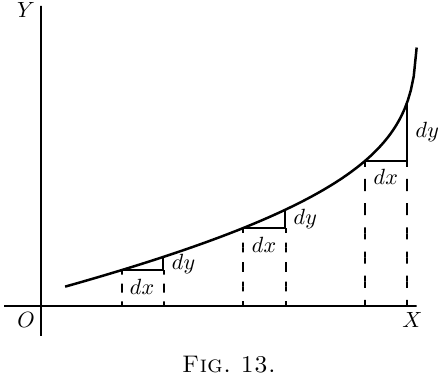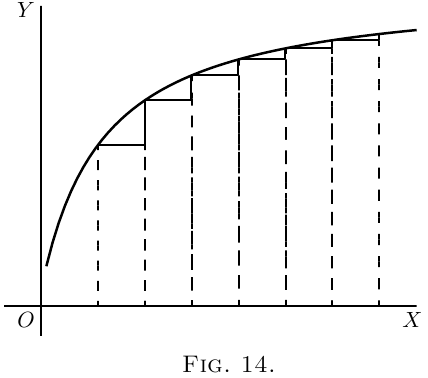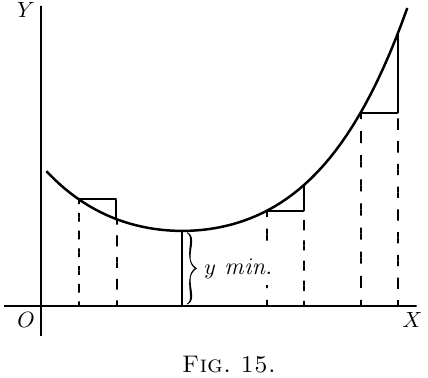If a curve first descends, and then goes up again, as in Figure 15, presenting a concavity upwards, then clearly $\dfrac{dy}{dx}$ will first be negative, with diminishing values as the curve flattens, then will be zero at the point where the bottom of the trough of the curve is reached; and from this point onward $\dfrac{dy}{dx}$ will have positive values that go on increasing. In such a case $y$ is said to pass by a minimum. The minimum value of $y$ is not necessarily the smallest value of $y$, it is that value of $y$ corresponding to the bottom of the trough; for instance, in Figure 28 (the value of $y$ corresponding to the bottom of the trough is $1$, while $y$ takes elsewhere values which are smaller than this. The characteristic of a minimum is that $y$ must increase on either side of it.

Note–For the particular value of $x$ that makes $y$ a minimum, the value of $\dfrac{dy}{dx} = 0$.

If a curve first ascends and then descends, the values of $\dfrac{dy}{dx}$ will be positive at first; then zero, as the summit is reached; then negative, as the curve slopes downwards, as in Figure 16. In this case $y$ is said to pass by a maximum, but the maximum value of $y$ is not necessarily the greatest value of $y$. In Figure 28, the maximum of $y$ is $2\frac{1}{3}$, but this is by no means the greatest value $y$ can have at some other point of the curve.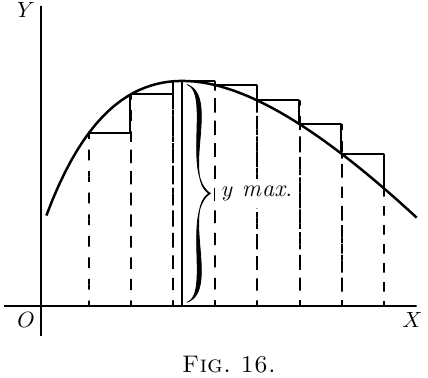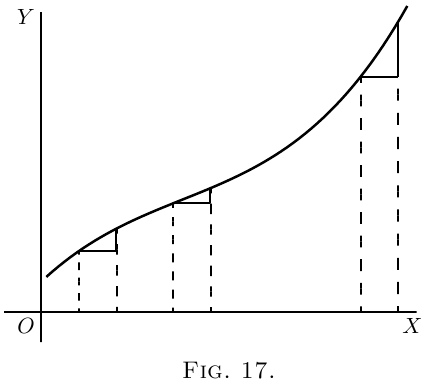Note–For the particular value of $x$ that makes $y$ a maximum, the value of $\dfrac{dy}{dx}= 0$.

If a curve has the peculiar form of Figure 17, the values of $\dfrac{dy}{dx}$ will always be positive; but there will be one particular place where the slope is least steep, where the value of $\dfrac{dy}{dx}$ will be a minimum; that is, less than it is at any other part of the curve.

If a curve has the form of Figure 18, the value of $\dfrac{dy}{dx}$ will be negative in the upper part, and positive in the lower part; while at the nose of the curve where it becomes actually perpendicular, the value of $\dfrac{dy}{dx}$ will be infinitely great.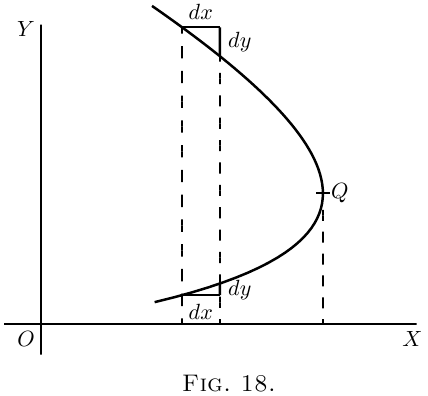Now that we understand that $\dfrac{dy}{dx}$ measures the steepness of a curve at any point, let us turn to some of the equations which we have already learned how to differentiate.

It is plotted out in Figure 19, using equal scales for $x$ and $y$. If we put $x = 0$, then the corresponding ordinate will be $y = b$; that is to say, the “curve” crosses the $y$-axis at the height $b$. From here it ascends at $45°$; for whatever values we give to $x$ to the right, we have an equal $y$ to ascend. The line has a gradient of $1$ in $1$.

Now differentiate $y = x+b$, by the rules we have already learned (here and here), and we get $\dfrac{dy}{dx} = 1$.

The slope of the line is such that for every little step $dx$ to the right, we go an equal little step $dy$ upward. And this slope is constant–always the same slope.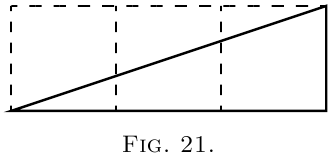(3) Now for a slightly harder case. \begin{align*} Let y= ax^2 + b. \end{align*}

Again the curve will start on the $y$-axis at a height $b$ above the origin.

Now differentiate. [If you have forgotten, turn back to here; or, rather, don't turn back, but think out the differentiation.] $\frac{dy}{dx} = 2ax.$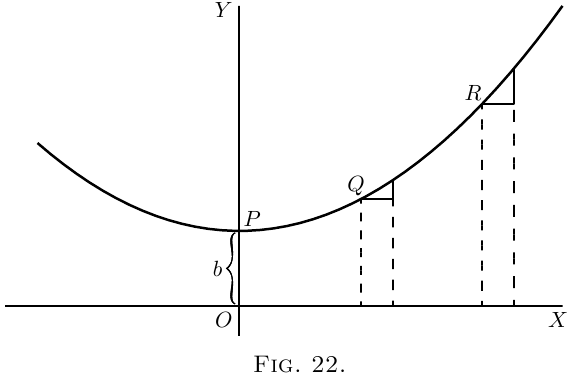This shows that the steepness will not be constant: it increases as $x$ increases. At the starting point $P$, where $x = 0$, the curve (Figure 22) has no steepness–that is, it is level. On the left of the origin, where $x$ has negative values, $\dfrac{dy}{dx}$ will also have negative values, or will descend from left to right, as in the Figure.

Let us illustrate this by working out a particular instance. Taking the equation $y = \tfrac{1}{4}x^2 + 3,$ and differentiating it, we get $\dfrac{dy}{dx} = \tfrac{1}{2}x.$ Now assign a few successive values, say from $0$ to $5$, to $x$; and calculate the corresponding values of $y$ by the first equation; and of $\dfrac{dy}{dx}$ from the second equation. Tabulating results, we have:

 $x$ $0$ $1$ $2$ $3$ $4$ $5$ $y$ $3$ $3\frac{1}{4}$ $4$ $5\frac{1}{4}$ $7$ $9\frac{1}{4}$ $d$ $0$ $\frac{1}{2}$ $1$ $1\frac{1}{2}$ $2$ $2\frac{1}{2}$
Then plot them out in two curves, in Figure 23 plotting the values of $y$ against those of $x$ and in Figure 24 those of $\dfrac{dy}{dx}$ against those of $x$. For any assigned value of $x$, the height of the ordinate in the second curve is proportional to the slope of the first curve.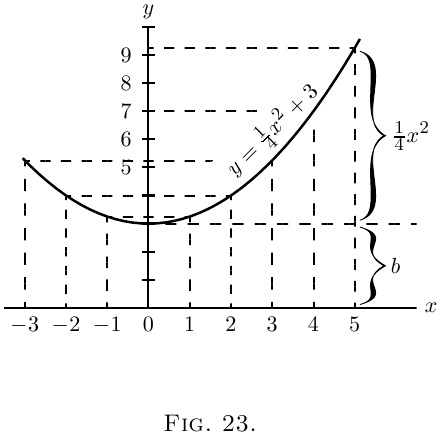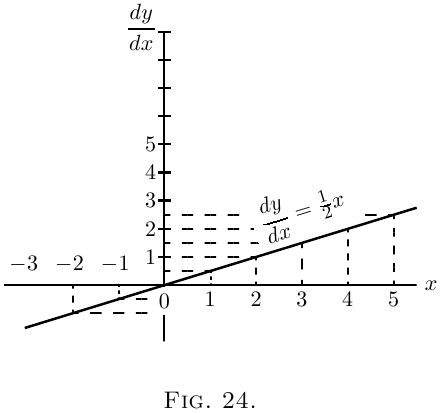If a curve comes to a sudden cusp, as in Figure 25, the slope at that point suddenly changes from a slope upward to a slope downward. In that case $\dfrac{dy}{dx}$ will clearly undergo an abrupt change from a positive to a negative value.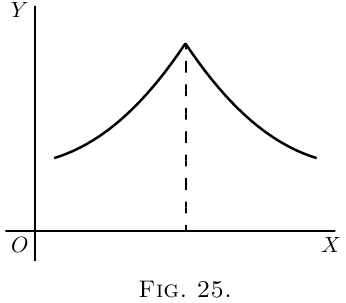The following examples show further applications of the principles just explained.

(4) Find the slope of the tangent to the curve $y = \frac{1}{2x} + 3,$ at the point where $x = -1$. Find the angle which this tangent makes with the curve $y = 2x^2 + 2$.

The slope of the tangent is the slope of the curve at the point where they touch one another (see here); that is, it is the $\dfrac{dy}{dx}$ of the curve for that point. Here $\dfrac{dy}{dx} = -\dfrac{1}{2x^2}$ and for $x = -1$, $\dfrac{dy}{dx} = -\dfrac{1}{2}$, which is the slope of the tangent and of the curve at that point. The tangent, being a straight line, has for equation $y = ax + b$, and its slope is $\dfrac{dy}{dx} = a$, hence $a = -\dfrac{1}{2}$. Also if $x= -1$, $y = \dfrac{1}{2(-1)} + 3 = 2\frac{1}{2}$; and as the tangent passes by this point, the coordinates of the point must satisfy the equation of the tangent, namely $y = -\dfrac{1}{2} x + b,$ so that $2\frac{1}{2} = -\dfrac{1}{2} × (-1) + b$ and $b = 2$; the equation of the tangent is therefore $y = -\dfrac{1}{2} x + 2$.

Now, when two curves meet, the intersection being a point common to both curves, its coordinates must satisfy the equation of each one of the two curves; that is, it must be a solution of the system of simultaneous equations formed by coupling together the equations of the curves. Here the curves meet one another at points given by the solution of \begin{aligned} y &= 2x^2 + 2, \\ y &= -\tfrac{1}{2} x + 2 \quad\text{or}\quad 2x^2 + 2 = -\tfrac{1}{2} x + 2; \end{aligned} that is, $x(2x + \tfrac{1}{2}) = 0.$

This equation has for its solutions $x = 0$ and $x = -\tfrac{1}{4}$. The slope of the curve $y = 2x^2 + 2$ at any point is $\dfrac{dy}{dx} = 4x.$

For the point where $x = 0$, this slope is zero; the curve is horizontal. For the point where $x = -\dfrac{1}{4},\quad \dfrac{dy}{dx} = -1;$ hence the curve at that point slopes downwards to the right at such an angle $\theta$ with the horizontal that $\tan \theta = 1$; that is, at $45°$ to the horizontal.

The slope of the straight line is $-\tfrac{1}{2}$; that is, it slopes downwards to the right and makes with the horizontal an angle $\phi$ such that $\tan \phi = \tfrac{1}{2}$; that is, an angle of $26° 34'$. It follows that at the first point the curve cuts the straight line at an angle of $26° 34'$, while at the second it cuts it at an angle of $45° - 26° 34' = 18° 26'$.

(5) A straight line is to be drawn, through a point whose coordinates are $x = 2$, $y = -1$, as tangent to the curve $y = x^2 - 5x + 6$. Find the coordinates of the point of contact.

The slope of the tangent must be the same as the $\dfrac{dy}{dx}$ of the curve; that is, $2x - 5$.

The equation of the straight line is $y = ax + b$, and as it is satisfied for the values $x = 2$, $y = -1$, then $-1 = a×2 + b$; also, its $\dfrac{dy}{dx} = a = 2x - 5$.

The $x$ and the $y$ of the point of contact must also satisfy both the equation of the tangent and the equation of the curve.

We have then \begin{aligned} y &= x^2 - 5x + 6, \,\,\,\,\,(i) \\ \end{aligned} \begin{aligned} y &= ax + b, \,\,\,\,\,(ii) \\ \end{aligned} \begin{aligned} -1 &= 2a + b, \,\,\,\,\,(iii) \\ \end{aligned} \begin{aligned} a &= 2x - 5, \,\,\,\,\,(iv) \end{aligned} four equations in $a$, $b$, $x$, $y$.

Equations (i) and (ii) give $x^2 - 5x + 6 = ax+b$.

Replacing $a$ and $b$ by their value in this, we get $x^2 - 5x + 6 = (2x - 5)x - 1 - 2(2x - 5),$ which simplifies to $x^2 - 4x + 3 = 0$, the solutions of which are: $x = 3$ and $x = 1$. Replacing in (i), we get $y = 0$ and $y = 2$ respectively; the two points of contact are then $x = 1$, $y = 2$, and $x = 3$, $y = 0$.

Note.–In all exercises dealing with curves, students will find it extremely instructive to verify the deductions obtained by actually plotting the curves.

### Exercises VIII

(1) Plot the curve $y = \tfrac{3}{4} x^2 - 5$, using a scale of millimetres. Measure at points corresponding to different values of $x$, the angle of its slope.

Find, by differentiating the equation, the expression for slope; and see, from a Table of Natural Tangents, whether this agrees with the measured angle.

(2) Find what will be the slope of the curve $y = 0.12x^3 - 2,$ at the particular point that has as abscissa $x = 2$.

(3) If $y = (x - a)(x - b)$, show that at the particular point of the curve where $\dfrac{dy}{dx} = 0$, $x$ will have the value $\tfrac{1}{2} (a + b)$.

(4) Find the $\dfrac{dy}{dx}$ of the equation $y = x^3 + 3x$; and calculate the numerical values of $\dfrac{dy}{dx}$ for the points corresponding to $x = 0$, $x = \tfrac{1}{2}$, $x = 1$, $x = 2$.

(5) In the curve to which the equation is $x^2 + y^2 = 4$, find the values of $x$ at those points where the slope ${} = 1$.

(6) Find the slope, at any point, of the curve whose equation is $\dfrac{x^2 }{3^2} + \dfrac{y^2}{2^2} = 1$; and give the numerical value of the slope at the place where $x = 0$, and at that where $x = 1$.

(7) The equation of a tangent to the curve $y = 5 - 2x + 0.5x^3$, being of the form $y = mx + n$, where $m$ and $n$ are constants, find the value of $m$ and $n$ if the point where the tangent touches the curve has $x=2$ for abscissa.

(8) At what angle do the two curves $y = 3.5x^2 + 2 \quad \text{and} \quad y = x^2 - 5x + 9.5$ cut one another?

(9) Tangents to the curve $y = ± \sqrt{25-x^2}$ are drawn at points for which $x = 3$ and $x = 4$. Find the coordinates of the point of intersection of the tangents and their mutual inclination.

(10) A straight line $y = 2x - b$ touches a curve $y = 3x^2 + 2$ at one point. What are the coordinates of the point of contact, and what is the value of $b$?

(2) $1.44$.

(4) $\dfrac{dy}{dx} = 3x^2 + 3$; and the numerical values are: $3$, $3 \frac{3}{4}$, $6$, and $15$.

(5) $± \sqrt{2}$.

(6) $\dfrac{dy}{dx} = - \dfrac{4}{9} \dfrac{x}{y}$. Slope is zero where $x = 0$; and is $\mp \dfrac{1}{3 \sqrt{2}}$ where $x = 1$.

(7) $m = 4$, $n = -3$.

(8) Intersections at $x = 1$, $x = -3$. Angles $153°\;26'$, $2°\;28'$.

(9) Intersection at $x = 3.57$, $y = 3.50$. Angle $16°\;16'$.

(10) $x = \frac{1}{3}$, $y = 2 \frac{1}{3}$, $b = -\frac{5}{3}$.

Next →
Main Page ↑